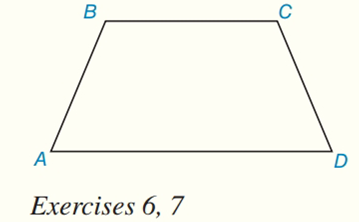Chapter 8.CR, Problem 6CRElementary Geometry For College St...

7th Edition
Alexander + 2 others
ISBN: 9781337614085

Solutions

Chapter
SectionElementary Geometry For College St...

7th Edition
Alexander + 2 others
ISBN: 9781337614085
Textbook Problem

Given: Trapezoid ABCD, with A B ¯ ≅ C D ¯ ,   B C = 6 , A D = 12 ,  and  A B = 5 Find: A A B C DTo determine

To find:

AABCD

Explanation

Theorem:

The area A of a trapezoid whose bases have lengths b1 and b2 and whose altitude has length h is given by

A=12h(b1+b2)

Calculation:

Consider the trapezoid ABCD with AB¯CD¯, BC=6,AD=12, and AB=5

The figure is shown below:

Still sussing out bartleby?

Check out a sample textbook solution.

See a sample solution

The Solution to Your Study Problems

Bartleby provides explanations to thousands of textbook problems written by our experts, many with advanced degrees!

Get Started

In Exercises 5-8, find the limit. limx01x(x+x)

Calculus: An Applied Approach (MindTap Course List)

Find f. f(x) = x6 4x4 + x + 1

Single Variable Calculus: Early Transcendentals

Sometimes, Always, or Never: limxaf(x)g(x)=limxaf(x)limxag(x).

Study Guide for Stewart's Single Variable Calculus: Early Transcendentals, 8th